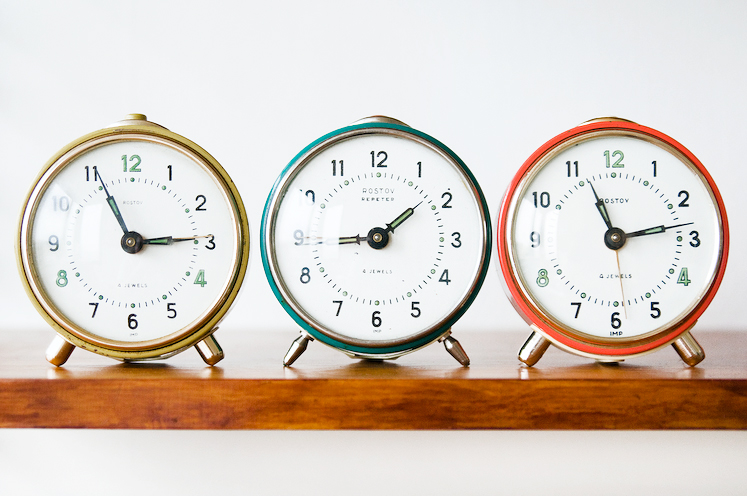# What a big difference a small change can makeI have three analog clocks. Clock 1 is correct, Clock 2 gains one minute every hour, and Clock 3 loses one minute every hour. I set them all to the correct time at $12$ AM. At $8 \! : \! 20$ PM, the angle between the hour and minute hands on Clock 1 is $A^{\circ}$, the angle between the hour and minute hands on Clock 2 is $B^{\circ}$, and the angle between the hour and minute hands on Clock 3 is $C^{\circ}$. Assume $0 \leq A, B, C \leq 180$. Find the value of $\left \lfloor A + B + C \right \rfloor$.

×